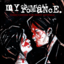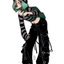MathematicsOpenStudy (anonymous):

is anyone going to help me plzzOpenStudy (anonymous):

step 1. Choose one of the groups of equations from the table below. Indicate which group you choose in your word processing file. Group 1 y = negative three-halvesx + 4 –3x + 2y = 8 Group 2 y = negative 2 over 3x – 2 –2x + 3y = -6 Group 3 y = negative 1 over 3x + 3 –x + 3y = 9 Group 4 y = negative 1 over 6x + 5 –x + 6y = 30 step 2. Type the first equation from the group you selected and identify the slope and the y-intercept of the equation. step 3. Describe how you would graph this line using the slope-intercept method. Be sure to write in complete sentences. step 4. Type the second equation from the group you selected and change the equation into slope-intercept form. Be sure to show all of your work. Identify the slope and y-intercept of the equation. step 5.Describe how you would graph this line using the slope-intercept method. Be sure to write in complete sentences. step 6. In a paragraph of at least three sentences, explain how the graphs of the two equations in your group are the same and how they are different. Be sure to use complete sentences.

Latest Questionshayhay6: Give an example of an equation of a line with an undefined slope. What type of li
8 minutes ago 1 Reply 0 Medalshayhay6: What is the equation of a line that passes through the point (5, -3) and has a sl
19 minutes ago 1 Reply 0 Medalspiperkoebel: Does anyone know the coordinates of two points on the line y = u22122?
21 minutes ago 1 Reply 0 Medalslolokrat: anyone good with microeconomics that can help me find out where i went wrong on m
11 hours ago 0 Replies 0 MedalsAsleepAndUnafraid21: URGENT PLZ HELP NOW Part C: Find the distance from B to E and from P to E. Show your work.
13 hours ago 49 Replies 5 MedalsKachirikutoka: How many solutions does the following equation have? |3x + 12| = 18 a No solution
17 hours ago 1 Reply 1 MedalAsleepAndUnafraid21: In the figure below, segment DE is parallel to segment BC and segment EF is parallel to AB: The figure shows triangle ABC with segments DE and DF.
14 hours ago 11 Replies 0 MedalsAsleepAndUnafraid21: In the figure below, segment DE is parallel to segment BC and segment EF is parallel to AB: The figure shows triangle ABC with segments DE and DF.
18 hours ago 1 Reply 0 Medalslakeawilliams: What is the solution to StartFraction 5 over 6 EndFraction x minus one-third grea
19 hours ago 1 Reply 0 Medalskendall22: Identify the sentence that uses modifiers in the correct form. A. The food looked great but tasted awfully.
19 hours ago 1 Reply 0 Medals